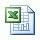Today, we would like to distribute the new update drilling formula version 1.3.

It is still totally FREE !!!.What new drilling formulas are added into the drilling formula version 1.3?

Effective Viscosity

Hydraulic Horse Power (HPP)

Optimum Flow Rate for basic system

Power Law Constant

Pressure Loss Annulus

Pressure Loss Annulus With Tool Joint Correction

Pressure Loss Drillstring

Pressure Loss Drillstring With Tool Joint Correction

Pressure Loss in Surface Equipment

Reynold Number

How many total useful drilling formulas in this version?

There are a total of 87 formulas which are divided into 7 categories (Applied Drilling Formulas, Basic Drilling Formulas, Directional Drilling Calculation, Drilling Fluid Formulas, Engineering Formulas, Hydraulic Formulas and Well Control Formulas).## Drilling Formula Calculation Sheet V1.1

I update some useful drilling formulas into the drilling formulas spread sheet called version 1.1.

## Click Here >>Drilling Formula Calculation Sheet V1.1

The following drilling formulas are added:

Calculate Influx Height

Hydrostatic Pressure Loss Due to Gas Cut Mud

Kill Weight Mud

Maximum pit gain from gas kick in water based mud

Maximum Surface Pressure from Gas Influx in Water Based Mud

You can read how to use this work sheet via this topic > Drilling Formula Calculation Sheet